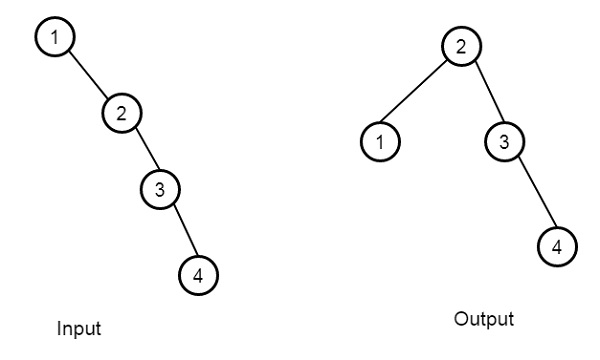# Balance a Binary Search Tree in c++

Suppose we have a binary search tree, we have to find a balanced binary search tree with the same node values. A binary search tree is said to be balanced if and only if the depth of the two subtrees of every node never differ by more than 1. If there is more than one result, return any of them. So if the tree is like −To solve this, we will follow these steps −

• Define inorder() method, this will store in order traversal sequence into an array

• Define the construct method(), this will take low and high −

• if low > high then return null

• mid := low + (high - low) / 2

• root := new node with value arr[mid]

• left of root := construct(low, mid – 1) and right of root := construct(mid + 1, high)

• return root

• From the main method, call the inorder method and return construct(0, size of arr - 1)

## Example (C++)

Let us see the following implementation to get a better understanding −

Live Demo

#include <bits/stdc++.h>
using namespace std;
class TreeNode{
public:
int val;
TreeNode *left, *right;
TreeNode(int data){
val = data;
left = right = NULL;
}
};
void insert(TreeNode **root, int val){
queue<TreeNode*> q;
q.push(*root);
while(q.size()){
TreeNode *temp = q.front();
q.pop();
if(!temp->left){
if(val != NULL)
temp->left = new TreeNode(val);
else
temp->left = new TreeNode(0);
return;
}else{
q.push(temp->left);
}
if(!temp->right){
if(val != NULL)
temp->right = new TreeNode(val);
else
temp->right = new TreeNode(0);
return;
}else{
q.push(temp->right);
}
}
}
TreeNode *make_tree(vector<int> v){
TreeNode *root = new TreeNode(v);
for(int i = 1; i<v.size(); i++){
insert(&root, v[i]);
}
return root;
}
void tree_level_trav(TreeNode*root){
if (root == NULL) return;
cout << "[";
queue<TreeNode *> q;
TreeNode *curr;
q.push(root);
q.push(NULL);
while (q.size() > 1) {
curr = q.front();
q.pop();
if (curr == NULL){
q.push(NULL);
} else {
if(curr->left)
q.push(curr->left);
if(curr->right)
q.push(curr->right);
if(curr->val == 0 || curr == NULL){
cout << "null" << ", ";
}else{
cout << curr->val << ", ";
}
}
}
cout << "]"<<endl;
}
class Solution {
public:
vector <int> arr;
void inorder(TreeNode* node){
if(!node || node->val == 0) return;
inorder(node->left);
arr.push_back(node->val);
inorder(node->right);
}
TreeNode* construct(int low, int high){
if(low > high) return NULL;
int mid = low + (high - low) / 2;
TreeNode* root = new TreeNode(arr[mid]);
root->left = construct(low, mid - 1);
root->right = construct(mid + 1, high);
return root;
}
TreeNode* balanceBST(TreeNode* root) {
inorder(root);
return construct(0, (int)arr.size() - 1);
}
};
main(){
vector<int> v = {1,NULL,2,NULL,NULL,NULL,3,NULL,NULL,NULL,NULL,NULL,NULL,NULL,4};
TreeNode *root = make_tree(v);
Solution ob;
tree_level_trav(ob.balanceBST(root));
}

## Input

[1,NULL,2,NULL,NULL,NULL,3,NULL,NULL,NULL,NULL,NULL,NULL,NULL,4]

## Output

[2, 1, 3, 4, ]